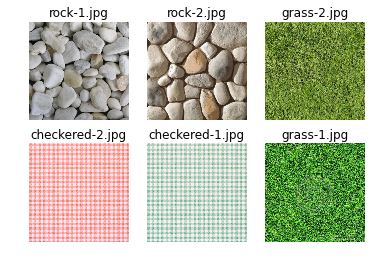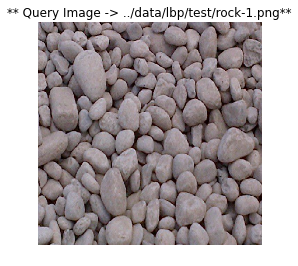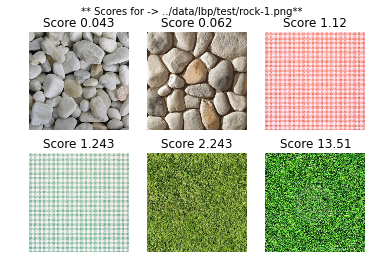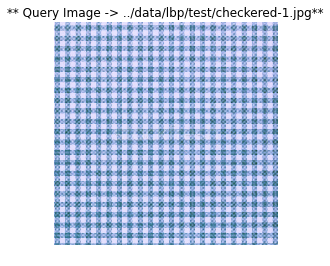# Texture Matching using Local Binary Patterns¶

Import the required modules.

In :
%pylab inline --no-import-all
# Utility package -- use pip install cvutils to install
import cvutils
# OpenCV bindings
import cv2
# To performing path manipulations
import os
# Local Binary Pattern function
from skimage.feature import local_binary_pattern
# To calculate a normalized histogram
from scipy.stats import itemfreq
from sklearn.preprocessing import normalize
# To read class from file
import csv

Populating the interactive namespace from numpy and matplotlib


Get the training set.

In :
# Store the path of training images in train_images
train_images = cvutils.imlist("../data/lbp/train/")
# Dictionary containing image paths as keys and corresponding label as value
train_dic = {}
with open('../data/lbp/class_train.txt', 'rb') as csvfile:
train_dic[row] = int(row)


Calculate the normalized LBP histograms for the training images.

In :
# List for storing the LBP Histograms, address of images and the corresponding label
X_test = []
X_name = []
y_test = []

# For each image in the training set calculate the LBP histogram
# and update X_test, X_name and y_test
for train_image in train_images:
# Convert to grayscale as LBP works on grayscale image
im_gray = cv2.cvtColor(im, cv2.COLOR_BGR2GRAY)
# Number of points to be considered as neighbourers
# Uniform LBP is used
lbp = local_binary_pattern(im_gray, no_points, radius, method='uniform')
# Calculate the histogram
x = itemfreq(lbp.ravel())
# Normalize the histogram
hist = x[:, 1]/sum(x[:, 1])
# Append image path in X_name
X_name.append(train_image)
# Append histogram to X_name
X_test.append(hist)
# Append class label in y_test
y_test.append(train_dic[os.path.split(train_image)])

# Display the testing images
nrows = 2
ncols = 3
fig, axes = plt.subplots(nrows,ncols)
for row in range(nrows):
for col in range(ncols):
axes[row][col].axis('off')
axes[row][col].set_title("{}".format(os.path.split(X_name[row*ncols+col])))Get the paths of the testing images.

In :
# Store the path of training images in test_images
test_images = cvutils.imlist("../data/lbp/test/")
# Dictionary containing image paths as keys and corresponding label as value
test_dic = {}
with open('../data/lbp/class_test.txt', 'rb') as csvfile:
test_dic[row] = int(row)


Read the testing images and for each image display the best 2 results. We compare the LBP Histograms of both the training images (calculated above) and testing images (calculated below) using Chi-Squared distance. We then sort the results based on the Chi-Squared distance and display the results in sorted order.

In :
for test_image in test_images:
# Convert to grayscale as LBP works on grayscale image
im_gray = cv2.cvtColor(im, cv2.COLOR_BGR2GRAY)
# Number of points to be considered as neighbourers
# Uniform LBP is used
lbp = local_binary_pattern(im_gray, no_points, radius, method='uniform')
# Calculate the histogram
x = itemfreq(lbp.ravel())
# Normalize the histogram
hist = x[:, 1]/sum(x[:, 1])
# Display the query image
cvutils.imshow("** Query Image -> {}**".format(test_image), im)
results = []
# For each image in the training dataset
# Calculate the chi-squared distance and the sort the values
for index, x in enumerate(X_test):
score = cv2.compareHist(np.array(x, dtype=np.float32), np.array(hist, dtype=np.float32), cv2.cv.CV_COMP_CHISQR)
results.append((X_name[index], round(score, 3)))
results = sorted(results, key=lambda score: score)
# Display the results
nrows = 2
ncols = 3
fig, axes = plt.subplots(nrows,ncols)
fig.suptitle("** Scores for -> {}**".format(test_image))
for row in range(nrows):
for col in range(ncols):